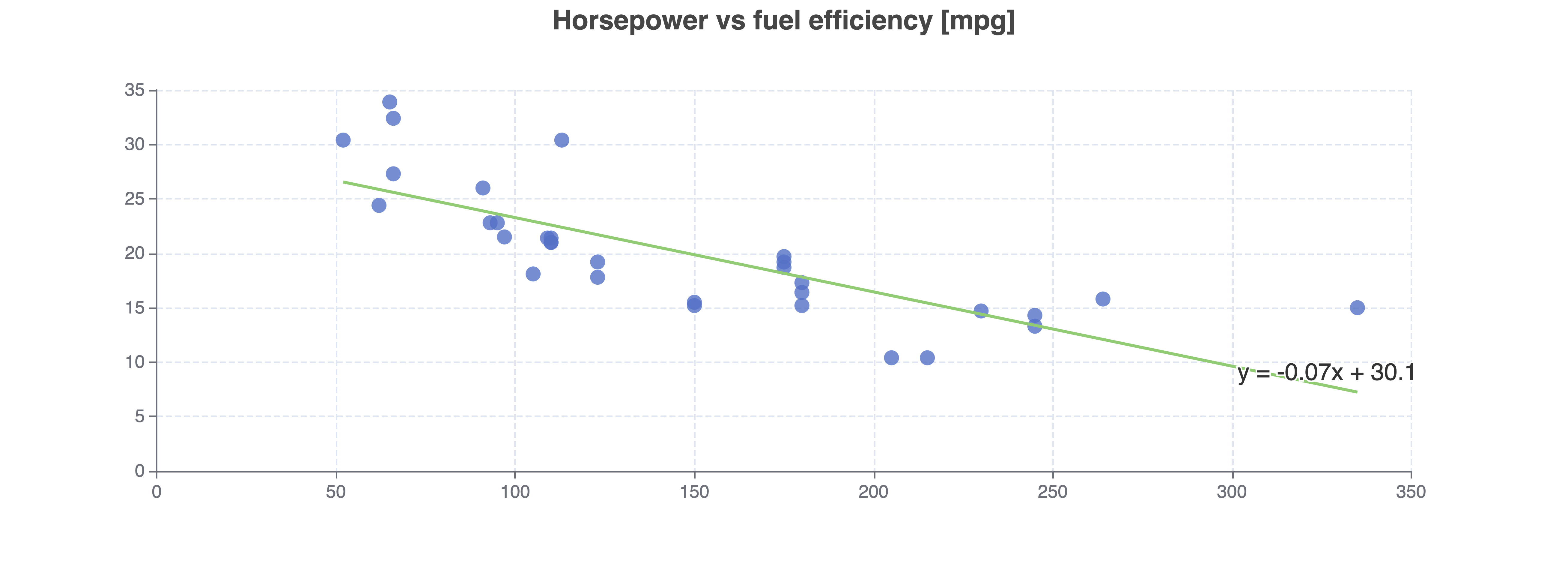# Linear Regression Calculator

Or input your data as csv

Sharing helps us build more free tools

Find a y = ax + b line of best fit with this free online linear regression calculator.

This linear regression calculator uses a straight line to model the relationship between two input variables.

Linear Regression is useful when there appears to be a straight-line relationship between your input variables. For example, the chart below shows how there is a linear relationship between horsepower and fuel efficiency for cars in the mtcars data set.## When To Use Linear Regression

This linear regression calculator is useful when you want to perform regression analysis and there appears to be a straight-line relationship between your input variables.

A scatter plot can be useful for taking a first look at the data for relationships.

The polynomial regression calculator is useful if the relationship appears to be a polynomial. The exponential regression calculator is useful if the relationship looks like an exponential curve.

## How To Perform Linear Regression

3. ### View regression fit

This page will calculate linear regression fit and show a regression line on the chart

## Linear Regression Equation

A linear regression model describes the relationship between a predictor (x) and a response variable (y) as a linear equation. Sometimes the predictor is called the independent variable and the response is called the dependent variable.

The first order simple linear regression equation looks like:Sometimes the gradient is called the slope coefficient and the intercept is called the intercept coefficient.

This linear regression calculator only calculates a linear line of best fit like the one above.

Linear regression models can also fit polynomials. E.g. the second order simple linear regression formula looks like:The regression line equation also generalizes to the nth power:This linear regression calculator does not calculate higher-order fits. To get an nth order fit use the polynomial regression calculator.

This linear regression calculator does not provide the r squared values of predictions yet. Please use the feedback form if you would like r squared values added.

## What is a Prediction Interval

Regression models provide an estimate for the y values given x values. Sometimes the uncertainty of the prediction can be modeled, this is called a prediction interval.

The prediction interval shows the range of y values that the model believes would occur for an x value. The interval is often stated as a confidence interval.

For example, the predicted value of y for a given x could be 10 with a 95% chance that it is between 8 and 12. The prediction interval is [8, 12].

## What Is the Difference Between Linear and Quadratic Regression

The difference between linear and quadratic regression depends on whether you are interested in the regression equation, or the shape of the line of best fit.

Fitting a quadratic line of best fit to input data is often considered quadratic regression.

The regression equation for fitting a quadratic function or a straight line is shown below.Notice how the predicted dependent variable y is made from a linear combination of the regression coefficients (the a's) and the predictor variable x. This means that the regression model for linear and quadratic regression is linear.

Statisticians consider both Linear and quadratic regression analysis to be linear because they both use a linear model to find the line of best fit.

## What are the Assumptions of Linear Regression

A Linear regression model makes four assumptions about the input data:

1. The relationship between the independent variable x and the dependent variable y is linear.
2. The variance of the residual of the fit model is the same for any value of x. This can be checked with a residual plot.
3. The input x, y data points are independent of each other
4. For any fixed value of the predictor x, the response y is normally distributed

## Linear Regression Prediction

After you have fit a model to input data, you can predict the value of new points. Prediction works best when the model fits the data well (r squared value close to 1) and the new data point is close to data points that were in your input data set.

To manually make a prediction without using a calculator you can pick a value on the regression line.

## Confidence Interval of the Prediction

Sometimes it is useful to know how confident the regression model is in its prediction. If the regression assumptions hold for the input data set, then it is possible to calculate a confidence interval for predictions.